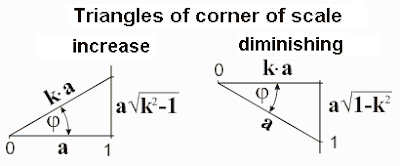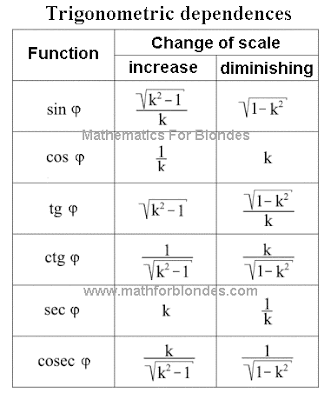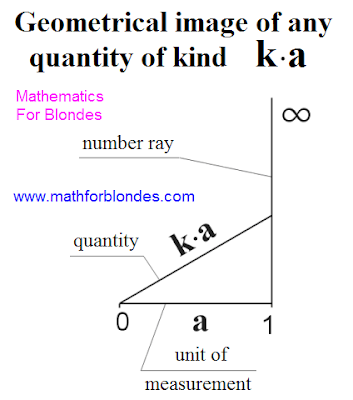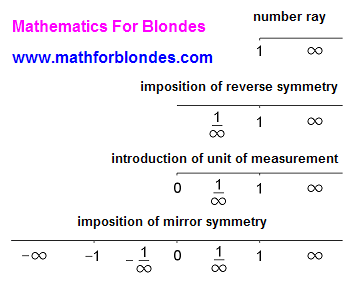## 10.26.2011

### Trigonometric dependences of corner of scale

We will consider trigonometric dependences of corner of scale in two rectangular triangles - at the increase of scale and at diminishing of scale.Trigonometric correlations of parties of the got rectangular triangles for diminishing and increase of scale we will take in a table.The got results can be compared to the relativistic radical from the theory of relativity of Einstein. Radicals in these equalizations very look like a sine and secant of diminishing of corner of scale. If to execute not difficult transformations, it is possible to get unit of measurement.The conducted transformations specify on that natural unit of measurement of speeds is speed of light. There are no grounds to suppose that there is a change of trigonometric dependences at passing through the point of symmetry of coefficient of scale. On it, it is possible to suppose that for faster-than-light speeds a relativistic radical takes on next values.On the basis of foregoing it is possible to suggest a next hypothesis about the structure of our Universe. Speed of set is a natural barrier separating slower-than-light part of Universe from faster-than-light part. Slower-than-light part of Universe we have possibility to look after. By virtue of specific properties of velocity of light, faster-than-light part of Universe can not be observed directly. It is possible to suppose that velocity of light is the axis of symmetry of distribution of substance in Universe. Dark matter and dark energy, that render affecting our part of Universe, can be in faster-than-light part of Universe.

If in Universe there are highly developed reasonable civilizations that captured faster-than-light technologies, then for an information transfer they will use not hertzian waves possessing velocity of light, and hard carriers of information on the basis of dark matter, transmissible with speeds, considerably excelling velocity of light.

If to equate trigonometric dependences of corner of scale with the values of trigonometric functions at 90° (it 1; 0 and 1/0), then for diminishing of scale they are taken to equality 0=1, for the increase of scale - to equality of k=0.

Our Universe has three limitations. In space by the border of universe явля-ется area, where velocity of light equals a zero. Outwardly our Universe is a point in space. Mathematical equalization of universe is equality 0=1 - any physical quantity with the unit of measurement in the scales of Universe equals a zero. This law of maintenance is confirmed by some researches of physicists, in particular, about it talked in the lecture of Andrei Linde.

Except spatial limitation, I exist limitations of speed. In slower-than-light part of Universe it is expressed in limitation long - distance between two positions of any point of space in time can not equal a zero. In physics this limitation it is accepted to name the absolute pitch of temperature. Faster-than-light part of Universe has limitation at times - time between two positions of any point in space can not equal a zero. The instantaneous moving in space without moving in time is impossible. Geometrically it can be expressed so: projection of speed on length and for a time can not equal a zero. Implementation of these terms is provided by the presence of rotation on the most different levels: atomic, planetary, galactic. It is possible to suppose that our Universe is similarly revolved in space.

More exact idea about principles of existence of Universe it is possible to get after the detailed study velocities of light as a physical process. For basis it is possible to accept position that velocity of light in our Universe is the result of co-operation of three dimension length with three dimension of time. Mathematically this co-operation is described by an multiplication. Physically our Universe has six dimension - three dimension of length and three dimension of time.

Expl for blondes: Now time to draw our Universe to look, what place she occupies in the space evolution.

## 10.25.2011

### Change of corner of scale

The increase of corner of scale can be presented as a change of quantity at unchanging unit of measurement. Diminishing of corner of scale can be presented as a change of unit of measurement at an unchanging quantity. Geometrically in the system of rectangular triangle it will look like the following.An increase and diminishing of scale in the identical amount of one times correspond to one value of corner of scale. On this property of corners of scale trigonometric dependences are based in a rectangular triangle.

From the point of view of mathematical result does not matter, as a change of quantity is described in relation to unit of measurement. A variable quantity at permanent unit of measurement and variable unit of measurement at a permanent quantity will give the identical value of corner of scale.

In a general view the change of scale of quantity can be presented as a turn of unit of measurement on the size of corner of scale. An increase or diminishing of scale depends on that, what position of unit of measurement is taken for basis at comparison and from the type of projection. The increase of scale can be presented as a radial projection on a number ray, perpendicular to the unit of measurement taken for basis at comparison. Diminishing of scale can be presented as a perpendicular projection of the compared unit of measurement on the unit of measurement taken for basis.Expl for blondes: A boring entry is farther closed and interesting begins are trigonometric dependences of corner of scale.

## 10.19.2011

### Quantity as basis of mathematics

Co-operation of numbers and units of measurements takes place in a point "unit" and expressed by a mathematical action by an multiplication. Geometrically unit of measurement is perpendicular to the numerical ray. The result of multiplication of numbers on unit of measurement in future will be named "quantity". All quantities are identical mathematical characteristics initially.All units of measurements in the surrounding us world it is possible to depict two methods: with a general point "unit" and with a general point "zero". The method of image does not influence on properties of making elements.

If to take unit for a general point, then this will be a circumference with a numerical ray going out the center of circumference. The radiuses of circumference will be units of measurements.Approximately it is so possible to represent any universe with all present in her units of measurements. The image of all units of measurements as radiuses of circumference underlines a that circumstance, that all units of measurements are identical mathematical characteristics initially. (Expl for blondes: And you does not it seem to that this portrait of mathematics very reminds the ancient invention of man - wheel? Are you exactly sure that a wheel was invented exactly by a man? Maybe, did someone try to explain to the man, what mathematics, but he so nothing and did not understand? In memory of meeting with the unknown teachers of mathematics history left us only a wheel... Why did unknown teachers begin the story exactly with it? Because not knowing and not understanding such elementary things, understanding to the mathematician is practically impossible.That the previous generations of mathematicians were brilliantly demonstrated us.)Approximately it is so possible to represent the moment of appearance of universe, that it is accepted to name "Big Bang". In this case a numerical ray graphicly can be presented as a numerical cone.

In an algebraic kind any quantity can be presented by multiplying of coefficient of scale by unit of measurement. Numbers come forward as a coefficient of scale.Geometrically any quantity that is the result of multiplication of coefficient of scale on unit of measurement, it is possible to present as a hypotenuse of rectangular triangle the cathetuses of that are unit of measurement and part of numerical ray.If the coefficient of scale is equal to unit, then a size is equal to unit of measurement.

Expl for blondes: Here now we got to one of types of mathematical corners we will consider and farther, what corner of scale and as there is a change of corner of scale.

## 10.18.2011

### Units of measurements and mathematical actions

Symmetry of addition and deduction in relation to a point a zero testifies that these mathematical actions can be executed only with one unit of measurement. Actually, addition and deduction reflect comparison of three numbers - two present and result. For different unitsof measurements, getting the result of these mathematical actions not maybe, as numbers have different warrants, and their comparison is not possible. The geometrical mapping of addition and deduction will be considered additionally.

Symmetry of multiplication and division in relation to a point "unit" allow to present dividing as multiplying by a number reverse to any number:

а : b = a x 1/b

Just, multiplying by a number reverse to any number, it is possible to present as dividing by any number:

а х 1/b = a : b

Traditional determination of prime fractional number as a result of division of two integers of p and q interchangebly to the result of increase of integer of p on a number reverse to the integer of q:

p : q = p x 1/q

In further exposition term a "multiplication" will imply an increase and division in the generally accepted sense because of their complete symmetry and relativity of these concepts.

Multiplication is this co-operation of two different units of measurements at right angles in a point "zero". As a result of co-operation new unit of measurement appears with beginning in a point "zero", that causes the quality change of interactive units of measurements. A mathematical action opposite on sense to the multiplication is decomposition on factors. Decomposition is executed through trigonometric functions that can have numerical and not numerical (0 and 1/0) values. Simplest similarity of decomposition under a corner in 45 degrees - this square root. Decomposition and trigonometric functions are more detailed will be considered additionally.

An area (for example, area of rectangle) is a result of co-operation of two perpendicular units of measurements of length. The multiplication of parallel units of measurements is not possible (at the multiplication of lengths of two parallel parties of rectangle, measured in meters, it is possible to get meters square, but it is impossible to get an area). Mathematical properties of units of measurements will be considered additionally.

As in mathematics it is accepted to distinguish the separate sets of numbers that is partly included in a concept "Any number", it is at a desire possible to set forth mathematically exact determinations for some from them. For example:

unit and all numbers that can be got addition of units are named natural;

all numbers that can be got addition or deduction of units are named integers (at deduction of the same amount of units, that is present, numbers apply in a zero);

numbers being not whole are named a fractional.

Expl for blondes: Now a turn came to look, as numbers and units co-operate in mathematics. This piece I named quantity.

## 10.17.2011

### Relativity of concept is "Any Number"

For the receipt of numerical axis does not matter, what from numbers are taken for any number: positive anymore units, positive less than units negative anymore minus units or negative less than minus units. Imposition reverse and mirror symmetries on any of these groups of numbers results in the receipt of all row of the real numbers.

From the choice of group of numbers as any number the results of mathematical actions will depend are different combinations of increase or diminishing of any number as a result of concrete mathematical action. In a table below the possible variants of concept "Any number" are marked just as there are corresponding to them fragments of numerical axis in traditionally assumed an air. For evidentness the increase of any number is doubled by a sign "+", diminishing - by the sign of "-", corresponding cells are distinguished by a different color.As be obvious from a table, addition and deduction are mirror symmetric in relation to a point "zero". An increase and division are mirror symmetric relatively two points are points "unit" and points "zero", here reverse symmetry is mirror symmetric in relation to a point "zero". All reasoning about priority and secondaryness of mathematical actions are an error. Symmetry of mathematical actions is considered in the separate article.

Expl for blondes: Farther we will consider units of measurements and mathematical actions.

## 9.27.2011

### Number line

In mathematics it is accepted to represent numbers as a number line. We will consider transformation of number ray to the number line.

Reverse symmetry allows to get numbers less unit. As a point of reverse symmetry is unit, this symmetry does not depend on units of measurement. Reverse symmetry reflects relativity of concepts "greater than unit" and "less than unit" at comparison of two numbers. In case of comparison of two numbers without fail it is needed to accept one of these numbers as unit of measurement.

After introduction of unit of measurement we get the absolute system of coordinates for any unit of measurement. Unit of measurement on a picture is represented in the traditionally accepted variant - with imposition on the area of reverse numbers. At imposition of mirror symmetry the point of that is a zero, we enter negative numbers and get the relative system of coordinates. All enumerated transformations are represented on a picture below, where the sign of endlessness is mark any number.Expl for blondes: Farther we will consider relativity of concept "Any number".

## 9.20.2011

### Multiplication chartMultiplication chart, printable multiplication table, multiplication tables chart 1 to 10 for you from mathematics for blondes.

Look similarly The multiplication table 1 to 20.